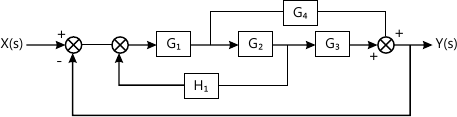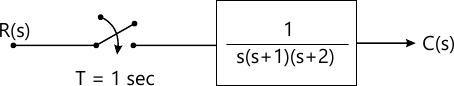Total marks: --
Total time: --
INSTRUCTIONS
(1) Assume appropriate data and state your reasons
(2) Marks are given to the right of every question
(3) Draw neat diagrams wherever necessary

Answer any one question from Q1 and Q2
1 (a) Explain open loop and closed loop systems with real time example.
6 M
1 (b) A unity feedback system has open loop transfer function: $G(s) = \dfrac {K} {s(s+10)}$ Determine 'K' so that damping factor is 0.5. For this value of 'K' determine:
(1) Location of closed loop poles,
(2) Peak overshoot, and
(3) Peak time. Assume input is unit step
6 M

2 (a) Find closed loop transfer function $\dfrac {y(s)}{x(s)} \ if \ G_1 = G_2 = \dfrac {1}{s+1} \ and \ G_3=G_4=s+1, \ H_1=1$ for system shown in Fig Q2 (a) using block diagram reduction technique.6 M
2 (b) If open loop transfer function is $G(s) = \dfrac {1}{s+1}$, obtain unit step response. Also find output at time t=0,1,2,3,4,5. Assume unity feedback and G(s) is in closed loop.
6 M

Answer any one question from Q3 and Q4
3 (a) Comment on stability using Routh criteria if characteristic equation is:
Q(s) = s5+2s4+3s3+4s2+5s+6=0
How many poles lie in right half of s-plane?
4 M
3 (b) Construct Nyquist plot and find phase frequency and gain margin if: $G(s)\cdot H(s) = \dfrac {1} {s(s+1)(s+2)}$ Also comment on stability.
8 M

4 (a) $If \ G(s) \ H(s)= \dfrac {k(s+2)}{s(s+1)(s+3)}.$ construct root locus and comment on stability of system.
7 M
4 (b) Obtain resonance peak and resonance frequency if: $G(s)\cdot H(s)= \dfrac {21} {s(s+5)} \ with \ H(s) =1$
4 M

5 (a) Obtain controllable and observable canonical state model if: $G(s) = \dfrac {y(s)}{u(s)} = \dfrac {s^3 + 2s^2 + 5s +1}{s^4 = 4s^3 + 4s^2 + 7s +2}$
6 M
Answer any one question from Q5 and Q6
5 (b) Find controllability and observability if: $A=\begin{bmatrix} -2 &1 &0 \\1 &-3 &2 \\10 &0 &-8 \end{bmatrix}, \ B=\begin{bmatrix} 0\\0.1 \\1 \end{bmatrix}, \ C=\begin{bmatrix} 1 &0 &1 \end{bmatrix}, \ D=$
7 M

6 (a) List advantages of state space over transfer function.
6 M
6 (b) Obtain state transition matrix if: $x= \begin{bmatrix} 0 &-3 \\1 &-4 \end{bmatrix} x(t)$
7 M

Answer any one question from Q7 and Q8
7 (a) Explain Ladder concept in PLC. Draw and explain different symbols used to construct ladder.
6 M
7 (b) Find pulse transfer function and impulse response for the system shown in Fig. Q. 7(b).7 M

8 (a) Write PID equation. For unit step input sketch the response of P, I, D action of PID.
6 M
8 (b) Write a note on digital control system with help of suitable block diagram.
7 M

More question papers from Control Systems